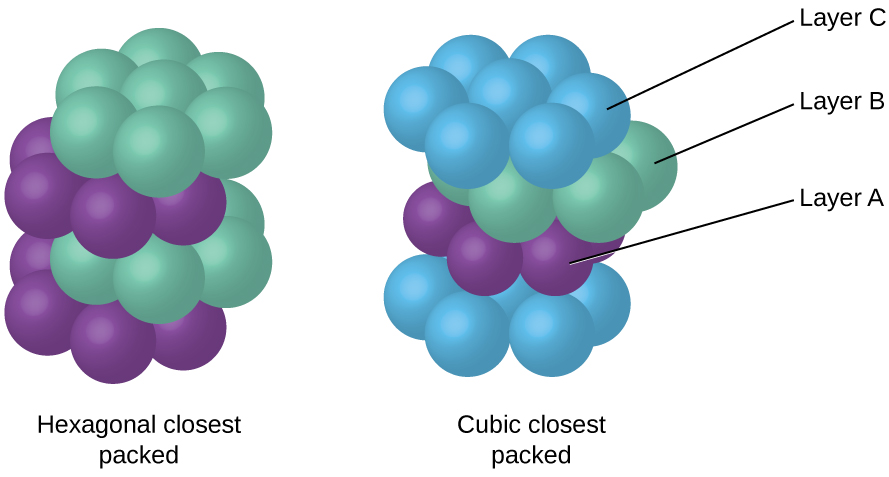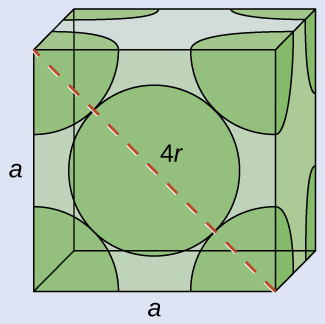# 10.6 Lattice structures in crystalline solids  (Page 4/29)

 Page 4 / 29

Because closer packing maximizes the overall attractions between atoms and minimizes the total intermolecular energy, the atoms in most metals pack in this manner. We find two types of closest packing in simple metallic crystalline structures: CCP, which we have already encountered, and hexagonal closest packing (HCP)    shown in [link] . Both consist of repeating layers of hexagonally arranged atoms. In both types, a second layer (B) is placed on the first layer (A) so that each atom in the second layer is in contact with three atoms in the first layer. The third layer is positioned in one of two ways. In HCP, atoms in the third layer are directly above atoms in the first layer (i.e., the third layer is also type A), and the stacking consists of alternating type A and type B close-packed layers (i.e., ABABAB⋯). In CCP, atoms in the third layer are not above atoms in either of the first two layers (i.e., the third layer is type C), and the stacking consists of alternating type A, type B, and type C close-packed layers (i.e., ABCABCABC⋯). About two–thirds of all metals crystallize in closest-packed arrays with coordination numbers of 12. Metals that crystallize in an HCP structure include Cd, Co, Li, Mg, Na, and Zn, and metals that crystallize in a CCP structure include Ag, Al, Ca, Cu, Ni, Pb, and Pt.In both types of closest packing, atoms are packed as compactly as possible. Hexagonal closest packing consists of two alternating layers (ABABAB…). Cubic closest packing consists of three alternating layers (ABCABCABC…).

## Calculation of atomic radius and density for metals, part 2

Calcium crystallizes in a face-centered cubic structure. The edge length of its unit cell is 558.8 pm.

(a) What is the atomic radius of Ca in this structure?

(b) Calculate the density of Ca.

## Solution(a) In an FCC structure, Ca atoms contact each other across the diagonal of the face, so the length of the diagonal is equal to four Ca atomic radii (d = 4 r ). Two adjacent edges and the diagonal of the face form a right triangle, with the length of each side equal to 558.8 pm and the length of the hypotenuse equal to four Ca atomic radii:

${a}^{2}+{a}^{2}={d}^{2}\phantom{\rule{0.2em}{0ex}}⟶\phantom{\rule{0.2em}{0ex}}{\left(558.8\phantom{\rule{0.2em}{0ex}}\text{pm}\right)}^{2}+{\left(558.5\phantom{\rule{0.2em}{0ex}}\text{pm}\right)}^{2}={\left(4r\right)}^{2}$

Solving this gives $r\phantom{\rule{0.2em}{0ex}}=\phantom{\rule{0.2em}{0ex}}\sqrt{\frac{{\left(558.8\phantom{\rule{0.2em}{0ex}}\text{pm}\right)}^{2}+{\left(558.5\phantom{\rule{0.2em}{0ex}}\text{pm}\right)}^{2}}{16}}\phantom{\rule{0.2em}{0ex}}=\text{197.6 pmg for a Ca radius}.$

(b) Density is given by $\text{density}\phantom{\rule{0.2em}{0ex}}=\phantom{\rule{0.2em}{0ex}}\frac{\text{mass}}{\text{volume}}.$ The density of calcium can be found by determining the density of its unit cell: for example, the mass contained within a unit cell divided by the volume of the unit cell. A face-centered Ca unit cell has one-eighth of an atom at each of the eight corners $\left(8\phantom{\rule{0.2em}{0ex}}×\phantom{\rule{0.2em}{0ex}}\frac{1}{8}\phantom{\rule{0.2em}{0ex}}=1$ atom) and one-half of an atom on each of the six faces $6\phantom{\rule{0.2em}{0ex}}×\phantom{\rule{0.2em}{0ex}}\frac{1}{2}\phantom{\rule{0.2em}{0ex}}=3$ atoms), for a total of four atoms in the unit cell.

The mass of the unit cell can be found by:

$\text{1 Ca unit cell}\phantom{\rule{0.2em}{0ex}}×\phantom{\rule{0.2em}{0ex}}\frac{\text{4 Ca atoms}}{\text{1 Ca unit cell}}\phantom{\rule{0.2em}{0ex}}×\phantom{\rule{0.2em}{0ex}}\frac{\text{1 mol Ca}}{6.022\phantom{\rule{0.2em}{0ex}}×\phantom{\rule{0.2em}{0ex}}{10}^{23}\phantom{\rule{0.2em}{0ex}}\text{Ca atoms}}\phantom{\rule{0.2em}{0ex}}×\phantom{\rule{0.2em}{0ex}}\frac{40.078\phantom{\rule{0.2em}{0ex}}\text{g}}{\text{1 mol Ca}}\phantom{\rule{0.2em}{0ex}}=2.662\phantom{\rule{0.2em}{0ex}}×\phantom{\rule{0.2em}{0ex}}{10}^{-22}\phantom{\rule{0.2em}{0ex}}\text{g}$

The volume of a Ca unit cell can be found by:

$V={a}^{3}={\left(558.8\phantom{\rule{0.2em}{0ex}}×\phantom{\rule{0.2em}{0ex}}{10}^{-10}\phantom{\rule{0.2em}{0ex}}\text{cm}\right)}^{3}=1.745\phantom{\rule{0.2em}{0ex}}×\phantom{\rule{0.2em}{0ex}}{10}^{-22}\phantom{\rule{0.2em}{0ex}}{\text{cm}}^{3}$

(Note that the edge length was converted from pm to cm to get the usual volume units for density.)

Then, the density of $\text{Po}\phantom{\rule{0.2em}{0ex}}=\phantom{\rule{0.2em}{0ex}}\frac{2.662\phantom{\rule{0.2em}{0ex}}×\phantom{\rule{0.2em}{0ex}}{10}^{-22}\phantom{\rule{0.2em}{0ex}}\text{g}}{1.745\phantom{\rule{0.2em}{0ex}}×\phantom{\rule{0.2em}{0ex}}{10}^{-22}\phantom{\rule{0.2em}{0ex}}{\text{cm}}^{3}}\phantom{\rule{0.2em}{0ex}}={\text{1.53 g/cm}}^{3}$

Silver crystallizes in an FCC structure. The edge length of its unit cell is 409 pm.

(a) What is the atomic radius of Ag in this structure?

(b) Calculate the density of Ag.

(a) 144 pm; (b) 10.5 g/cm 3

What is saturated hydrocarbon?
Alkane series are saturated Hydrocarbon
ok
hamza
it is hydrocarbon without double bond, like alkane
habibah
hydrocarbons that can no longer undergo additional reaction
what is the meaninh of link
Yes wish to join am a science ⚗ student
What is acid
Emmanuel
is agroup of substance that relaise hydrogen ion in the water solution.
mengistu
thanks
Aisha
An acid is a proton donor or an electron pair acceptor
amanda
how can someone understand chemistry vividly
Maybe by reading proofs or practical work and application in modern world.
Abdelkarim
what is isotopes
whats de shape of water
water has no shape because it's liquid
Wil
water is a shapeless, odourless, colourless and tasteless substance that only takes the shape of its container.
mikefred
i think they're referring to the molecular shape?
It has no shape but takes the shape of the container
Yeah I think so too and it's a bent shape
amanda
what isthe maening pkw
what is the meaning of STP
Laura
introduction to chemistry for beginner
hi
noble
through out human history
Yes?
Esther
hello
noble
yes
Bol
what
noble
is euglena a unicellular organ
is euglena a unicellular organism
Agio
how is hydrogen can be heated
what is difference between atom and molecule
Atom is the smallest part of matter; it consists of equal number of protons and electrons. It may have neutrons. A molecule is a compound made of atoms covalently bonded.
Abdelkarim
does amoeba has structure
what is the effect of green house on the earth 🌎
what is the effect of green house on the earth 🌎
kolawole
what's an atom?
it's the smallest unit of Matter
Orsine
atom
Eden
smallest part of an element
lydia
also, depending on its (atom's) structure, that is the amount of protons and neutrons and electrons, is the determining factors of what element it is.
Richard
is a smallast particals of an element
Buwembo
it is the smallest part of an element that can take part in a chemical reaction
Ayub
is the smallest part of an element
Jonathan
An atom is the smallest indivisible part of a matter

#### Get Jobilize Job Search Mobile App in your pocket Now!ByByBy Anh DaoBy OpenStaxBy OpenStaxBy RhodesBy P. Wynn NormanBy OpenStaxBy OpenStaxBy Stephanie RedfernBy Lakeima RobertsBy Wey Hey